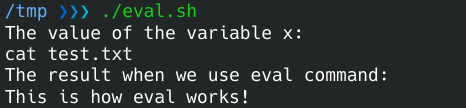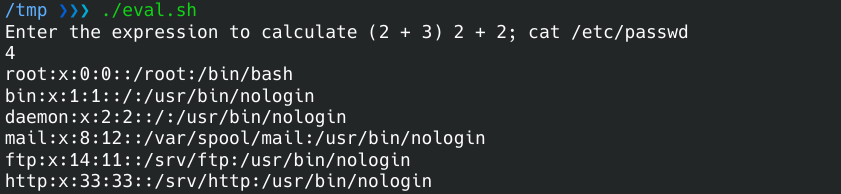Use Eval Command in Linux Bash

This article shows how to use the eval command in Linux Bash. Then, we will address the vulnerability that this command can cause.

Use the eval Command in Bash

The eval command interprets the given arguments as a Bash command.

Let’s take an example to understand better. We have a file, test.txt, containing This is how eval works!.

Below is the content of the example Bash script.

x="cat test.txt"
echo "The value of the variable x: "
echo \$x

echo "The result when we use eval command:"
eval \$x

First, we print the variable x with echo. This is exactly the value we defined.

Then, we use the eval command with the variable we defined. This time, the content of the variable is executed as a command, and the contents of test.txt get printed.Be Cautious When Using eval in Bash

The eval command is evil. Be careful when using this command.

Consider the following example of a simple Bash script that calculates the given expression and prints the result to the screen. Since the eval will execute the given input as a Bash command, this will also execute if the user provides a malicious command as an input.

read -p "Enter the expression to calculate (2 + 3) " expression
result="expr \$expression"
eval \$resultWrite for us
DelftStack articles are written by software geeks like you. If you also would like to contribute to DelftStack by writing paid articles, you can check the write for us page.